# Image compression Algorithms

30 de May de 2014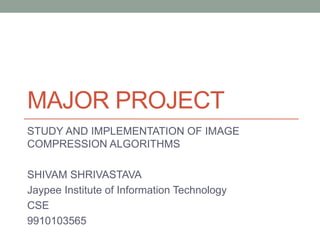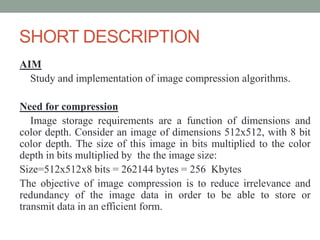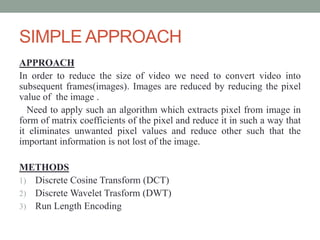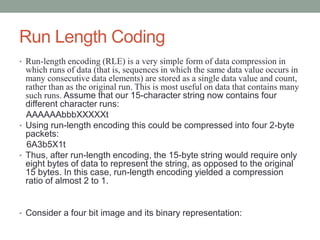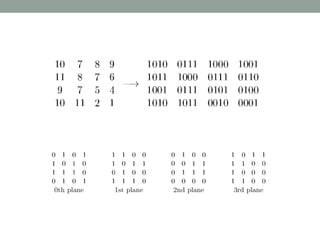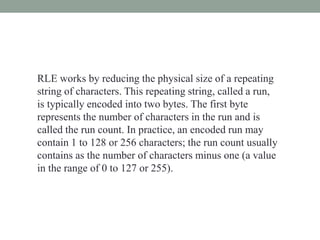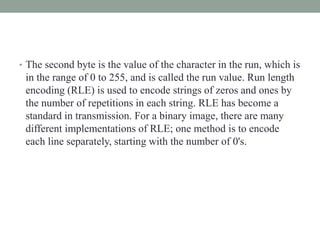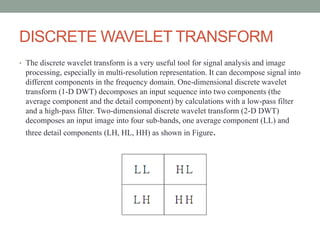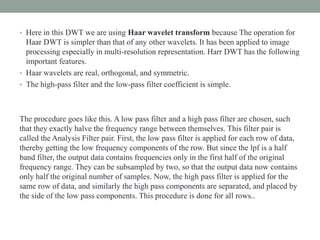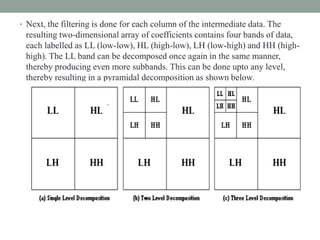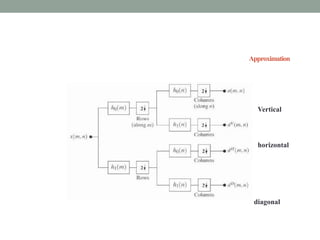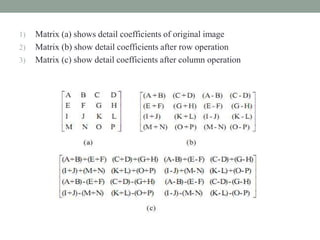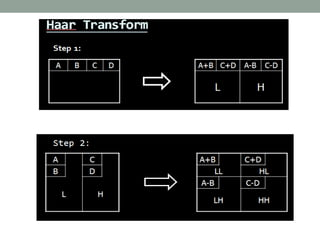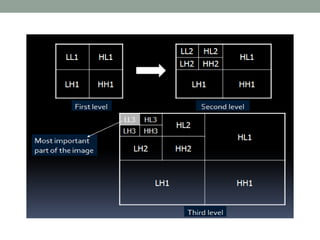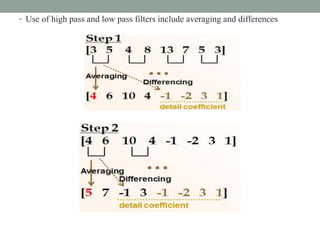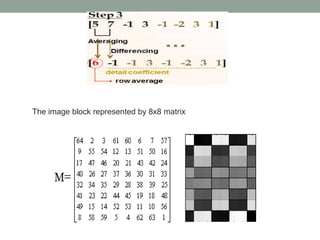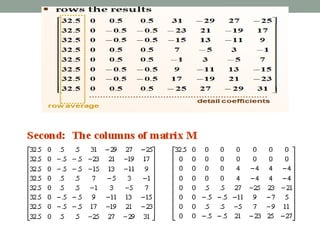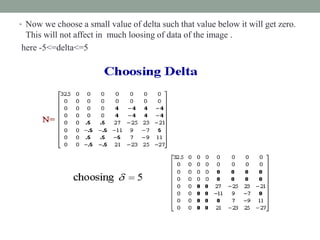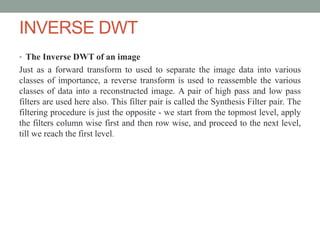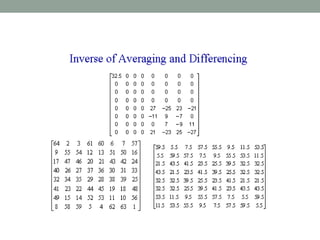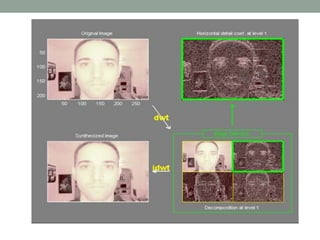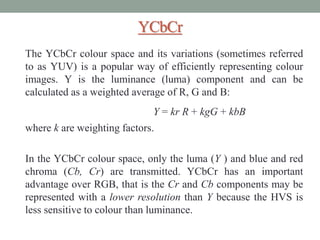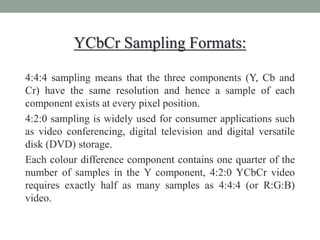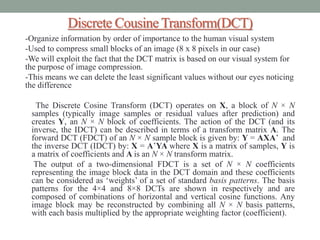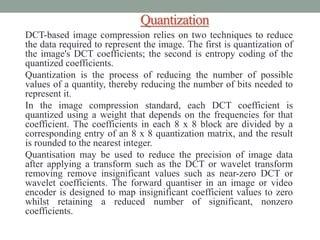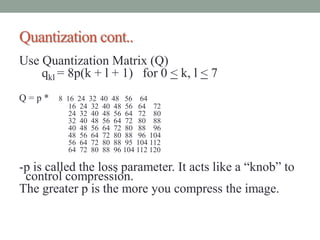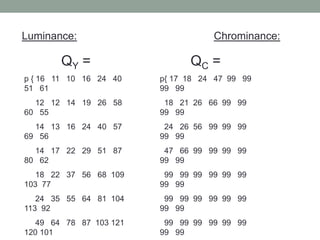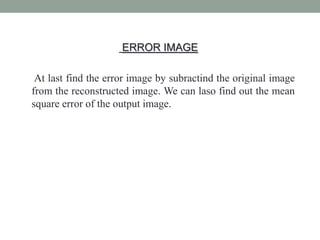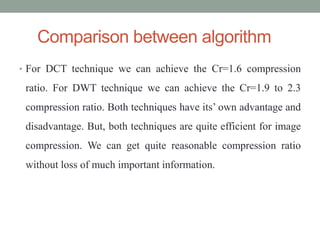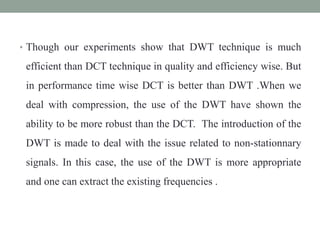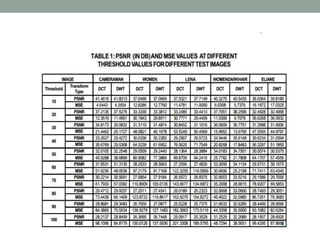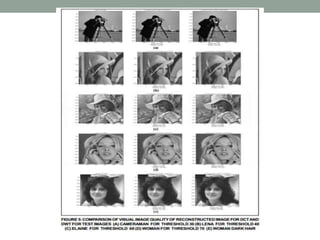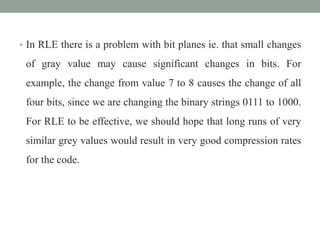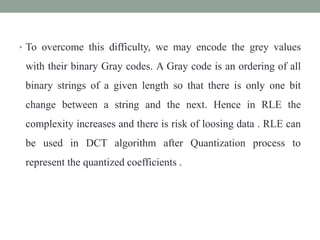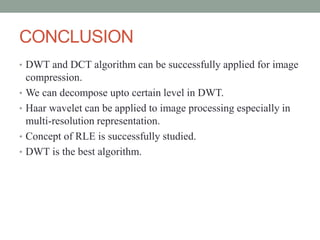1 de 35

### Image compression Algorithms

• 1. MAJOR PROJECT STUDY AND IMPLEMENTATION OF IMAGE COMPRESSION ALGORITHMS SHIVAM SHRIVASTAVA Jaypee Institute of Information Technology CSE 9910103565
• 2. SHORT DESCRIPTION AIM Study and implementation of image compression algorithms. Need for compression Image storage requirements are a function of dimensions and color depth. Consider an image of dimensions 512x512, with 8 bit color depth. The size of this image in bits multiplied to the color depth in bits multiplied by the the image size: Size=512x512x8 bits = 262144 bytes = 256 Kbytes The objective of image compression is to reduce irrelevance and redundancy of the image data in order to be able to store or transmit data in an efficient form.
• 3. SIMPLE APPROACH APPROACH In order to reduce the size of video we need to convert video into subsequent frames(images). Images are reduced by reducing the pixel value of the image . Need to apply such an algorithm which extracts pixel from image in form of matrix coefficients of the pixel and reduce it in such a way that it eliminates unwanted pixel values and reduce other such that the important information is not lost of the image. METHODS 1) Discrete Cosine Transform (DCT) 2) Discrete Wavelet Trasform (DWT) 3) Run Length Encoding
• 4. Run Length Coding • Run-length encoding (RLE) is a very simple form of data compression in which runs of data (that is, sequences in which the same data value occurs in many consecutive data elements) are stored as a single data value and count, rather than as the original run. This is most useful on data that contains many such runs. Assume that our 15-character string now contains four different character runs: AAAAAAbbbXXXXXt • Using run-length encoding this could be compressed into four 2-byte packets: 6A3b5X1t • Thus, after run-length encoding, the 15-byte string would require only eight bytes of data to represent the string, as opposed to the original 15 bytes. In this case, run-length encoding yielded a compression ratio of almost 2 to 1. • Consider a four bit image and its binary representation:
• 6. RLE works by reducing the physical size of a repeating string of characters. This repeating string, called a run, is typically encoded into two bytes. The first byte represents the number of characters in the run and is called the run count. In practice, an encoded run may contain 1 to 128 or 256 characters; the run count usually contains as the number of characters minus one (a value in the range of 0 to 127 or 255).
• 7. • The second byte is the value of the character in the run, which is in the range of 0 to 255, and is called the run value. Run length encoding (RLE) is used to encode strings of zeros and ones by the number of repetitions in each string. RLE has become a standard in transmission. For a binary image, there are many different implementations of RLE; one method is to encode each line separately, starting with the number of 0's.
• 8. DISCRETE WAVELET TRANSFORM • The discrete wavelet transform is a very useful tool for signal analysis and image processing, especially in multi-resolution representation. It can decompose signal into different components in the frequency domain. One-dimensional discrete wavelet transform (1-D DWT) decomposes an input sequence into two components (the average component and the detail component) by calculations with a low-pass filter and a high-pass filter. Two-dimensional discrete wavelet transform (2-D DWT) decomposes an input image into four sub-bands, one average component (LL) and three detail components (LH, HL, HH) as shown in Figure.
• 9. • Here in this DWT we are using Haar wavelet transform because The operation for Haar DWT is simpler than that of any other wavelets. It has been applied to image processing especially in multi-resolution representation. Harr DWT has the following important features. • Haar wavelets are real, orthogonal, and symmetric. • The high-pass filter and the low-pass filter coefficient is simple. The procedure goes like this. A low pass filter and a high pass filter are chosen, such that they exactly halve the frequency range between themselves. This filter pair is called the Analysis Filter pair. First, the low pass filter is applied for each row of data, thereby getting the low frequency components of the row. But since the lpf is a half band filter, the output data contains frequencies only in the first half of the original frequency range. They can be subsampled by two, so that the output data now contains only half the original number of samples. Now, the high pass filter is applied for the same row of data, and similarly the high pass components are separated, and placed by the side of the low pass components. This procedure is done for all rows..
• 10. • Next, the filtering is done for each column of the intermediate data. The resulting two-dimensional array of coefficients contains four bands of data, each labelled as LL (low-low), HL (high-low), LH (low-high) and HH (high- high). The LL band can be decomposed once again in the same manner, thereby producing even more subbands. This can be done upto any level, thereby resulting in a pyramidal decomposition as shown below.
• 11. Approximation Vertical horizontal diagonal
• 12. 1) Matrix (a) shows detail coefficients of original image 2) Matrix (b) show detail coefficients after row operation 3) Matrix (c) show detail coefficients after column operation
• 15. • Use of high pass and low pass filters include averaging and differences
• 16. The image block represented by 8x8 matrix
• 18. • Now we choose a small value of delta such that value below it will get zero. This will not affect in much loosing of data of the image . here -5<=delta<=5
• 19. INVERSE DWT • The Inverse DWT of an image Just as a forward transform to used to separate the image data into various classes of importance, a reverse transform is used to reassemble the various classes of data into a reconstructed image. A pair of high pass and low pass filters are used here also. This filter pair is called the Synthesis Filter pair. The filtering procedure is just the opposite - we start from the topmost level, apply the filters column wise first and then row wise, and proceed to the next level, till we reach the first level.
• 22. YCbCr The YCbCr colour space and its variations (sometimes referred to as YUV) is a popular way of efficiently representing colour images. Y is the luminance (luma) component and can be calculated as a weighted average of R, G and B: Y = kr R + kgG + kbB where k are weighting factors. In the YCbCr colour space, only the luma (Y ) and blue and red chroma (Cb, Cr) are transmitted. YCbCr has an important advantage over RGB, that is the Cr and Cb components may be represented with a lower resolution than Y because the HVS is less sensitive to colour than luminance.
• 23. YCbCr Sampling Formats: 4:4:4 sampling means that the three components (Y, Cb and Cr) have the same resolution and hence a sample of each component exists at every pixel position. 4:2:0 sampling is widely used for consumer applications such as video conferencing, digital television and digital versatile disk (DVD) storage. Each colour difference component contains one quarter of the number of samples in the Y component, 4:2:0 YCbCr video requires exactly half as many samples as 4:4:4 (or R:G:B) video.
• 24. Discrete Cousine Transform(DCT) -Organize information by order of importance to the human visual system -Used to compress small blocks of an image (8 x 8 pixels in our case) -We will exploit the fact that the DCT matrix is based on our visual system for the purpose of image compression. -This means we can delete the least significant values without our eyes noticing the difference The Discrete Cosine Transform (DCT) operates on X, a block of N × N samples (typically image samples or residual values after prediction) and creates Y, an N × N block of coefficients. The action of the DCT (and its inverse, the IDCT) can be described in terms of a transform matrix A. The forward DCT (FDCT) of an N × N sample block is given by: Y = AXA’ and the inverse DCT (IDCT) by: X = A’YA where X is a matrix of samples, Y is a matrix of coefficients and A is an N × N transform matrix. The output of a two-dimensional FDCT is a set of N × N coefficients representing the image block data in the DCT domain and these coefficients can be considered as ‘weights’ of a set of standard basis patterns. The basis patterns for the 4×4 and 8×8 DCTs are shown in respectively and are composed of combinations of horizontal and vertical cosine functions. Any image block may be reconstructed by combining all N × N basis patterns, with each basis multiplied by the appropriate weighting factor (coefficient).
• 25. Quantization DCT-based image compression relies on two techniques to reduce the data required to represent the image. The first is quantization of the image's DCT coefficients; the second is entropy coding of the quantized coefficients. Quantization is the process of reducing the number of possible values of a quantity, thereby reducing the number of bits needed to represent it. In the image compression standard, each DCT coefficient is quantized using a weight that depends on the frequencies for that coefficient. The coefficients in each 8 x 8 block are divided by a corresponding entry of an 8 x 8 quantization matrix, and the result is rounded to the nearest integer. Quantisation may be used to reduce the precision of image data after applying a transform such as the DCT or wavelet transform removing remove insignificant values such as near-zero DCT or wavelet coefficients. The forward quantiser in an image or video encoder is designed to map insignificant coefficient values to zero whilst retaining a reduced number of significant, nonzero coefficients.
• 26. Quantization cont.. Use Quantization Matrix (Q) qkl = 8p(k + l + 1) for 0 < k, l < 7 Q = p * 8 16 24 32 40 48 56 64 16 24 32 40 48 56 64 72 24 32 40 48 56 64 72 80 32 40 48 56 64 72 80 88 40 48 56 64 72 80 88 96 48 56 64 72 80 88 96 104 56 64 72 80 88 95 104 112 64 72 80 88 96 104 112 120 -p is called the loss parameter. It acts like a “knob” to control compression. The greater p is the more you compress the image.
• 27. Luminance: Chrominance: QY = p { 16 11 10 16 24 40 51 61 12 12 14 19 26 58 60 55 14 13 16 24 40 57 69 56 14 17 22 29 51 87 80 62 18 22 37 56 68 109 103 77 24 35 55 64 81 104 113 92 49 64 78 87 103 121 120 101 QC = p{ 17 18 24 47 99 99 99 99 18 21 26 66 99 99 99 99 24 26 56 99 99 99 99 99 47 66 99 99 99 99 99 99 99 99 99 99 99 99 99 99 99 99 99 99 99 99 99 99 99 99 99 99 99 99 99 99
• 28. ERROR IMAGE At last find the error image by subractind the original image from the reconstructed image. We can laso find out the mean square error of the output image.
• 29. Comparison between algorithm • For DCT technique we can achieve the Cr=1.6 compression ratio. For DWT technique we can achieve the Cr=1.9 to 2.3 compression ratio. Both techniques have its’ own advantage and disadvantage. But, both techniques are quite efficient for image compression. We can get quite reasonable compression ratio without loss of much important information.
• 30. • Though our experiments show that DWT technique is much efficient than DCT technique in quality and efficiency wise. But in performance time wise DCT is better than DWT .When we deal with compression, the use of the DWT have shown the ability to be more robust than the DCT. The introduction of the DWT is made to deal with the issue related to non-stationnary signals. In this case, the use of the DWT is more appropriate and one can extract the existing frequencies .
• 33. • In RLE there is a problem with bit planes ie. that small changes of gray value may cause significant changes in bits. For example, the change from value 7 to 8 causes the change of all four bits, since we are changing the binary strings 0111 to 1000. For RLE to be effective, we should hope that long runs of very similar grey values would result in very good compression rates for the code.
• 34. • To overcome this difficulty, we may encode the grey values with their binary Gray codes. A Gray code is an ordering of all binary strings of a given length so that there is only one bit change between a string and the next. Hence in RLE the complexity increases and there is risk of loosing data . RLE can be used in DCT algorithm after Quantization process to represent the quantized coefficients .
• 35. CONCLUSION • DWT and DCT algorithm can be successfully applied for image compression. • We can decompose upto certain level in DWT. • Haar wavelet can be applied to image processing especially in multi-resolution representation. • Concept of RLE is successfully studied. • DWT is the best algorithm.